# Class Activation Map methods implemented in Pytorch

`pip install grad-cam`

Tested on many Common CNN Networks and Vision Transformers.

Includes smoothing methods to make the CAMs look nice.

Full support for batches of images in all methods.Method What it does
AblationCAM Zero out activations and measure how the output drops (this repository includes a fast batched implementation)
ScoreCAM Perbutate the image by the scaled activations and measure how the output drops
EigenCAM Takes the first principle component of the 2D Activations (no class discrimination, but seems to give great results)
EigenGradCAM Like EigenCAM but with class discrimination: First principle component of Activations*Grad. Looks like GradCAM, but cleaner
LayerCAM Spatially weight the activations by positive gradients. Works better especially in lower layers

### Combining Grad-CAM with Guided Backpropagation for the 'pug, pug-dog' class:# More Visual Examples

#### Swin Transfomer (Tiny window:7 patch:4 input-size:224):

It seems that GradCAM++ is almost the same as GradCAM, in most networks except VGG where the advantage is larger.

# Chosing the Target Layer

You need to choose the target layer to compute CAM for. Some common choices are:

• Resnet18 and 50: model.layer4[-1]
• VGG and densenet161: model.features[-1]
• mnasnet1_0: model.layers[-1]
• ViT: model.blocks[-1].norm1
• SwinT: model.layers[-1].blocks[-1].norm1

# Using from code as a library

```from pytorch_grad_cam import GradCAM, ScoreCAM, GradCAMPlusPlus, AblationCAM, XGradCAM, EigenCAM
from torchvision.models import resnet50

model = resnet50(pretrained=True)
target_layer = model.layer4[-1]
input_tensor = # Create an input tensor image for your model..
# Note: input_tensor can be a batch tensor with several images!

# Construct the CAM object once, and then re-use it on many images:

# If target_category is None, the highest scoring category
# will be used for every image in the batch.
# target_category can also be an integer, or a list of different integers
# for every image in the batch.
target_category = 281

# You can also pass aug_smooth=True and eigen_smooth=True, to apply smoothing.
grayscale_cam = cam(input_tensor=input_tensor, target_category=target_category)

# In this example grayscale_cam has only one image in the batch:
grayscale_cam = grayscale_cam[0, :]
visualization = show_cam_on_image(rgb_img, grayscale_cam)```

# Smoothing to get nice looking CAMs

To reduce noise in the CAMs, and make it fit better on the objects, two smoothing methods are supported:

• `aug_smooth=True`

Test time augmentation: increases the run time by x6.

Applies a combination of horizontal flips, and mutiplying the image by [1.0, 1.1, 0.9].

This has the effect of better centering the CAM around the objects.

• `eigen_smooth=True`

First principle component of `activations*weights`

This has the effect of removing a lot of noise.

# Running the example script:

Usage: `python cam.py --image-path <path_to_image> --method <method>`

To use with CUDA: `python cam.py --image-path <path_to_image> --use-cuda`

You can choose between:

`GradCAM` , `ScoreCAM`, `GradCAMPlusPlus`, `AblationCAM`, `XGradCAM` , `LayerCAM` and `EigenCAM`.

Some methods like ScoreCAM and AblationCAM require a large number of forward passes, and have a batched implementation.

You can control the batch size with `cam.batch_size = `

# How does it work with Vision Transformers

See usage_examples/vit_example.py

In ViT the output of the layers are typically BATCH x 197 x 192. In the dimension with 197, the first element represents the class token, and the rest represent the 14x14 patches in the image. We can treat the last 196 elements as a 14x14 spatial image, with 192 channels.

To reshape the activations and gradients to 2D spatial images, we can pass the CAM constructor a reshape_transform function.

This can also be a starting point for other architectures that will come in the future.

```GradCAM(model=model, target_layer=target_layer, reshape_transform=reshape_transform)

def reshape_transform(tensor, height=14, width=14):
result = tensor[:, 1 :  , :].reshape(tensor.size(0),
height, width, tensor.size(2))

# Bring the channels to the first dimension,
# like in CNNs.
result = result.transpose(2, 3).transpose(1, 2)
return result```

### Which target_layer should we chose for Vision Transformers?

Since the final classification is done on the class token computed in the last attention block, the output will not be affected by the 14x14 channels in the last layer. The gradient of the output with respect to them, will be 0!

We should chose any layer before the final attention block, for example:

`target_layer = model.blocks[-1].norm1`

# How does it work with Swin Transformers

See usage_examples/swinT_example.py

In Swin transformer base the output of the layers are typically BATCH x 49 x 1024. We can treat the last 49 elements as a 7x7 spatial image, with 1024 channels.

To reshape the activations and gradients to 2D spatial images, we can pass the CAM constructor a reshape_transform function.

This can also be a starting point for other architectures that will come in the future.

```GradCAM(model=model, target_layer=target_layer, reshape_transform=reshape_transform)

def reshape_transform(tensor, height=7, width=7):
result = tensor.reshape(tensor.size(0),
height, width, tensor.size(2))

# Bring the channels to the first dimension,
# like in CNNs.
result = result.transpose(2, 3).transpose(1, 2)
return result```

### Which target_layer should we chose for Swin Transformers?

Since the swin transformer is different from ViT, it does not contains `cls_token` as present in ViT, therefore we will use all the 7x7 images we get from the last block of the last layer.

We should chose any layer before the final attention block, for example:

`target_layer = model.layers[-1].blocks[-1].norm1`

## Citation

If you use this for research, please cite. Here is an example BibTeX entry:

``````@misc{jacobgilpytorchcam,
title={PyTorch library for CAM methods},
author={Jacob Gildenblat and contributors},
year={2021},
publisher={GitHub},
}
``````

# References

https://arxiv.org/abs/1610.02391
`Grad-CAM: Visual Explanations from Deep Networks via Gradient-based Localization Ramprasaath R. Selvaraju, Michael Cogswell, Abhishek Das, Ramakrishna Vedantam, Devi Parikh, Dhruv Batra`

https://arxiv.org/abs/1710.11063
`Grad-CAM++: Improved Visual Explanations for Deep Convolutional Networks Aditya Chattopadhyay, Anirban Sarkar, Prantik Howlader, Vineeth N Balasubramanian`

https://arxiv.org/abs/1910.01279
`Score-CAM: Score-Weighted Visual Explanations for Convolutional Neural Networks Haofan Wang, Zifan Wang, Mengnan Du, Fan Yang, Zijian Zhang, Sirui Ding, Piotr Mardziel, Xia Hu`

https://ieeexplore.ieee.org/abstract/document/9093360/
`Ablation-cam: Visual explanations for deep convolutional network via gradient-free localization. Saurabh Desai and Harish G Ramaswamy. In WACV, pages 972–980, 2020`

https://arxiv.org/abs/2008.02312
`Axiom-based Grad-CAM: Towards Accurate Visualization and Explanation of CNNs Ruigang Fu, Qingyong Hu, Xiaohu Dong, Yulan Guo, Yinghui Gao, Biao Li`

https://arxiv.org/abs/2008.00299
`Eigen-CAM: Class Activation Map using Principal Components Mohammed Bany Muhammad, Mohammed Yeasin`

http://mftp.mmcheng.net/Papers/21TIP_LayerCAM.pdf
`LayerCAM: Exploring Hierarchical Class Activation Maps for Localization Peng-Tao Jiang; Chang-Bin Zhang; Qibin Hou; Ming-Ming Cheng; Yunchao Wei`

• #### Add a conda installation option

Adding a conda installation option could be very helpful. I have started working on a PR (https://github.com/conda-forge/staged-recipes/pull/17244) already to add `grad-cam` from PyPI to conda-forge channel. Once the PR is merged, `grad-cam` could be installed as follows.

``````conda install -c conda-forge grad-cam
``````

:bulb: I will open a PR here to update the install instructions, once `grad-cam` is available on conda-forge channel.

opened by sugatoray 18
• #### AxisError: axis 2 is out of bounds for array of dimension 2

Getting the following error when trying out the cam function on an image example. This might be an issue with how I have loaded in my data, but not sure how to debug it.

Code to reproduce:

``````from pytorch_grad_cam import GradCAM, ScoreCAM, GradCAMPlusPlus, AblationCAM, XGradCAM, EigenCAM
import PIL

target_layers = [model.linear_layers[-1]]
img, label = img.to(DEVICE), label.to(DEVICE)

img = img.float()

target_category = None

grayscale_cam = cam(input_tensor=img)

grayscale_cam = grayscale_cam[0, :]
visualization = show_cam_on_image(rgb_img, grayscale_cam, use_rgb=True)
``````

Note the image is of shape `torch.Size([64, 1, 128, 128])` Here is the traceback:

``````---------------------------------------------------------------------------
AxisError                                 Traceback (most recent call last)
<ipython-input-188-441d284cf4e0> in <module>()
14 target_category = None
15
---> 16 grayscale_cam = cam(input_tensor=img)
17
18 grayscale_cam = grayscale_cam[0, :]

7 frames
/usr/local/lib/python3.7/dist-packages/pytorch_grad_cam/base_cam.py in __call__(self, input_tensor, targets, aug_smooth, eigen_smooth)
183
184         return self.forward(input_tensor,
--> 185                             targets, eigen_smooth)
186
187     def __del__(self):

/usr/local/lib/python3.7/dist-packages/pytorch_grad_cam/base_cam.py in forward(self, input_tensor, targets, eigen_smooth)
93         cam_per_layer = self.compute_cam_per_layer(input_tensor,
94                                                    targets,
---> 95                                                    eigen_smooth)
96         return self.aggregate_multi_layers(cam_per_layer)
97

/usr/local/lib/python3.7/dist-packages/pytorch_grad_cam/base_cam.py in compute_cam_per_layer(self, input_tensor, targets, eigen_smooth)
128                                      layer_activations,
--> 130                                      eigen_smooth)
131             cam = np.maximum(cam, 0)
132             scaled = scale_cam_image(cam, target_size)

52                                        targets,
53                                        activations,
55         weighted_activations = weights[:, :, None, None] * activations
56         if eigen_smooth:

20                         activations,
---> 22         return np.mean(grads, axis=(2, 3))

<__array_function__ internals> in mean(*args, **kwargs)

/usr/local/lib/python3.7/dist-packages/numpy/core/fromnumeric.py in mean(a, axis, dtype, out, keepdims)
3371
3372     return _methods._mean(a, axis=axis, dtype=dtype,
-> 3373                           out=out, **kwargs)
3374
3375

/usr/local/lib/python3.7/dist-packages/numpy/core/_methods.py in _mean(a, axis, dtype, out, keepdims)
145
146     is_float16_result = False
--> 147     rcount = _count_reduce_items(arr, axis)
148     # Make this warning show up first
149     if rcount == 0:

/usr/local/lib/python3.7/dist-packages/numpy/core/_methods.py in _count_reduce_items(arr, axis)
64     items = 1
65     for ax in axis:
---> 66         items *= arr.shape[mu.normalize_axis_index(ax, arr.ndim)]
67     return items
68

AxisError: axis 2 is out of bounds for array of dimension 2
``````
opened by asfandyarazhar13 16
• #### Doubt regarding ViT from timm

Hi @jacobgil. This is such an amazing piece of work. Thanks to you and all the contributors behind it.

I am currently, using `vit_base_patch16_224` from `timm` and I am trying to visualize the Grad-CAM maps. I have followed the guidelines you have laid out in the README for ViTs but I am still getting a weird error:

`RuntimeError: shape '[1, 16, 16, 768]' is invalid for input of size 150528`.

Here's minimal code:

``````def reshape_transform(tensor, height=14, width=14):
result = tensor[:, 1 :  , :].reshape(tensor.size(0),
height, width, tensor.size(2))

# Bring the channels to the first dimension,
# like in CNNs.
result = result.transpose(2, 3).transpose(1, 2)
return result

vit_model = timm.create_model("vit_base_patch16_224", pretrained=True)

rgb_img = cv2.imread("grace_hopper.jpg", 1)[:, :, ::-1]
rgb_img = cv2.resize(rgb_img, (224, 224))
rgb_img = np.float32(rgb_img) / 255
input_tensor = preprocess_image(rgb_img, mean=[0.485, 0.456, 0.406],
std=[0.229, 0.224, 0.225])

use_cuda=True, reshape_transform=reshape_transform)
grayscale_cam = cam(input_tensor=input_tensor, target_category=652)
visualization = show_cam_on_image(rgb_img, grayscale_cam)
``````

Here's the Colab Notebook for reproducing the issue.

opened by sayakpaul 14
• #### How can I use grad-cam in FPN net?

I have been using FPN network structure recently, but I have been unable to properly visualize with grad-cam.If anyone knows how to write code, please let me know.Thans a lot.

opened by ChrisHJC 14
• #### Problem visualizing cam on trained model

Hi, I am using this script to evaluate my results on a brand classification for cars. When I run this algoithm on the model in pytorch library (models.resnet34) pretrained on imagenet in which i just changed the classification head with:

`
input_size = model_resnet.fc.in_features

model_resnet.fc = nn.Sequential( nn.Linear(input_size, 256), nn.ReLU(), nn.Dropout(0.2), nn.Linear(256, num_classes), nn.Softmax(), ) ` the cam that I got as output actually make sense and look like this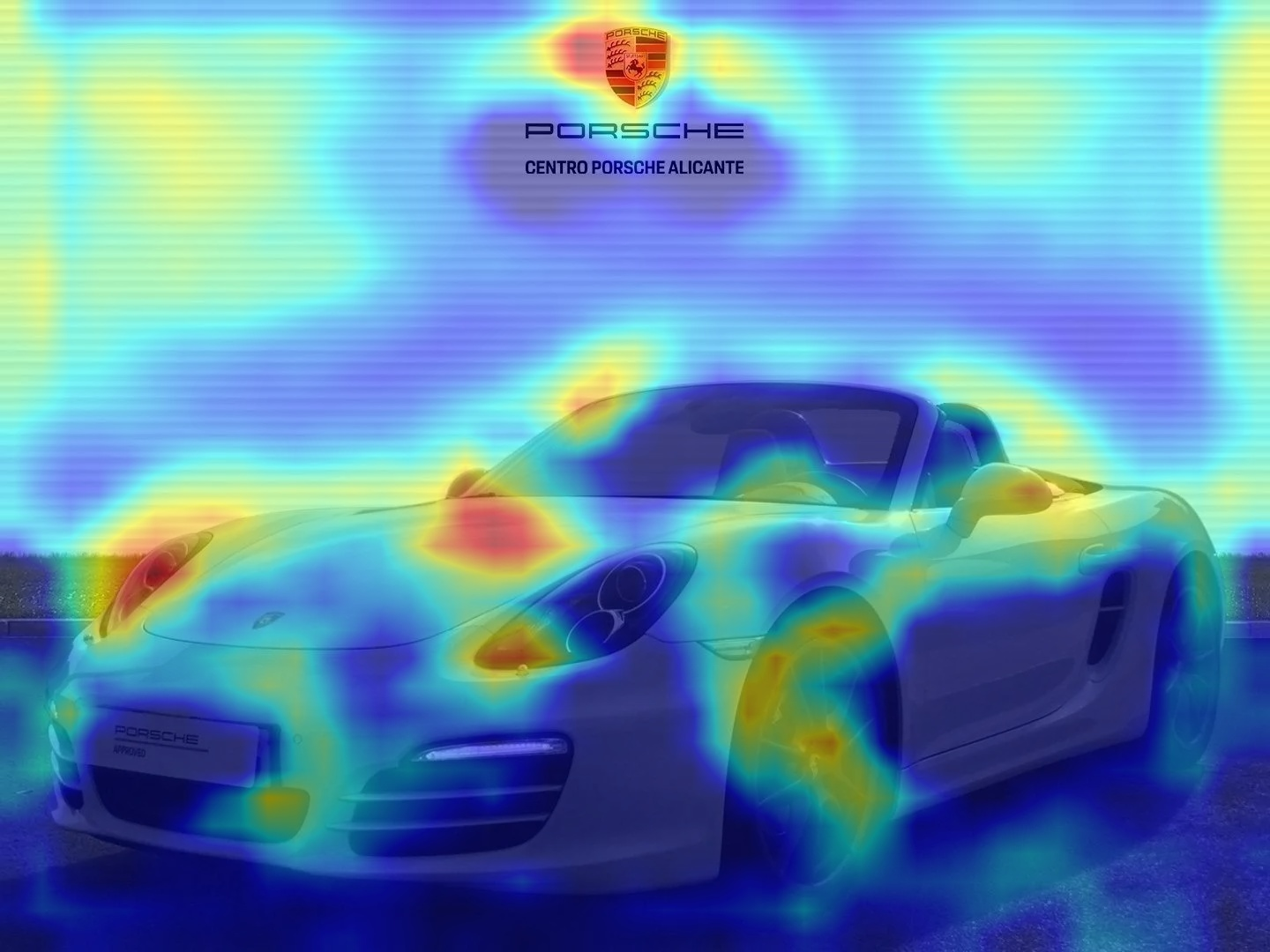While when i load the resnet34 with the new head (same structure as before), that I trained from scratch and that has an accuracy > 90% for each class, it gives me an activation map that doens't make sense and it's the same no matter what input image (given that it belongs to the same class).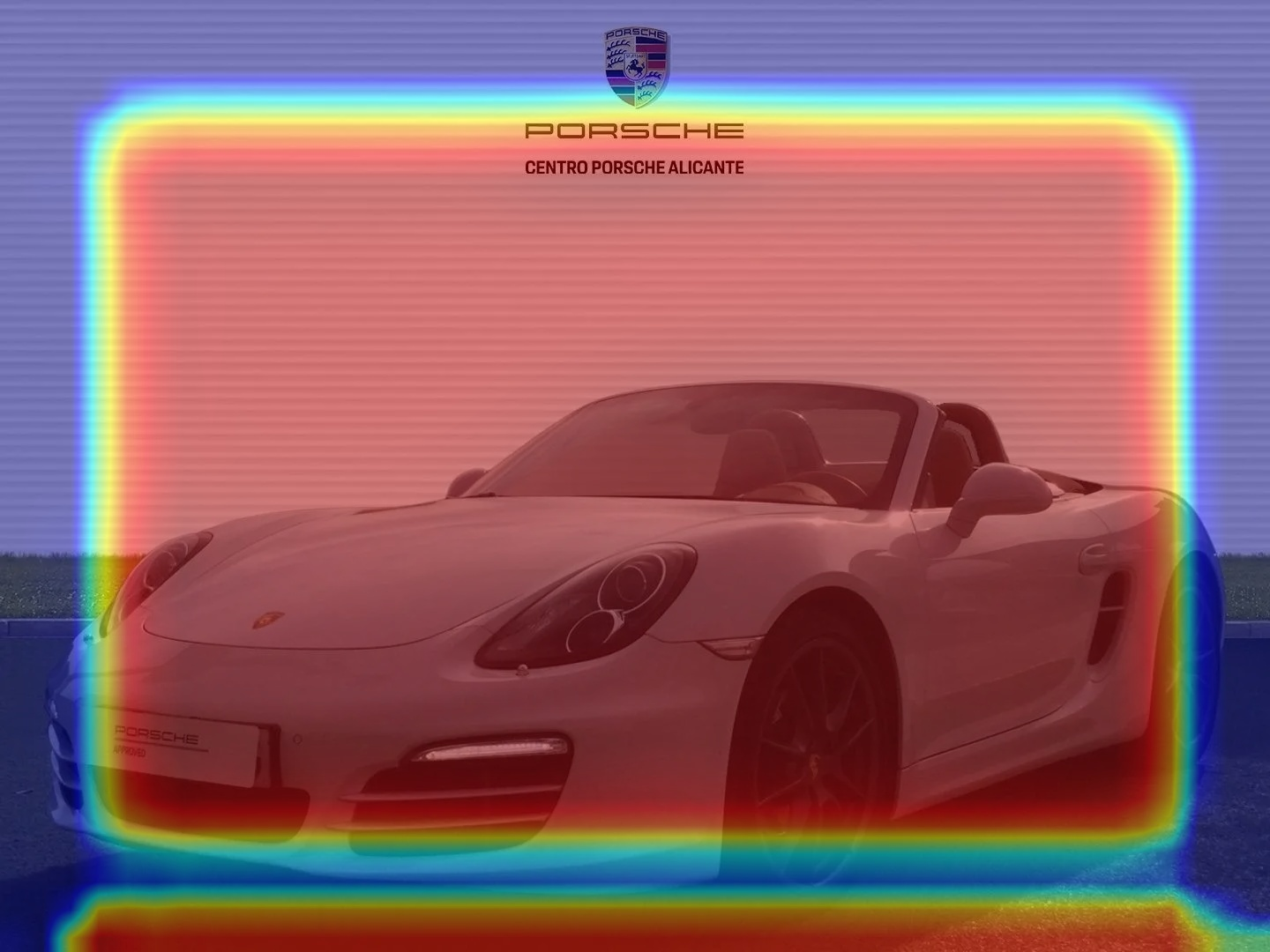I'm struggling to come up to an explanation but I don't understand it because the perfromance doesn't match the cam. I would be very gratefull if you have some advice.

opened by davcaste 13
• #### Can I use it for 3D models ? And what is the parameter target for these segmentation models?

I have a 3D Unet model that takes as input a 5D tensor of size (1,1,4,256,256) which is 4 frame video and outputs a 5D tensor (1,3,4,256,256) a video predicted mask for 3 labels .

Can I use the library or its not suitable for my segmentation problem ,in case I can use it what's the target parameter supposed to be .

opened by ReemShalaata 11
• #### element 0 of tensors does not require grad and does not have a grad_fn

When I learned your example to write code, my code did report such an error at runtime:

RuntimeError: element 0 of tensors does not require grad and does not have a grad_fn

How should we solve this problem？

opened by EatonL 11
• #### AxisError: axis 2 is out of bounds for array of dimension 0

I' currently facing a strange behaviour when using GradCam for my tuned model. When I Fine-tune it from scratch everything works fine, but when I do Feature Extraction I get the mentioned error.

My Script for the training:

``````print("PyTorch` Version: ",torch.__version__)
print("Torchvision Version: ",torchvision.__version__)

data_dir = "/content/bt_models/data/training/"

# Models to choose from [resnet, alexnet, vgg, squeezenet, densenet, inception]
model_name = "resnet"

# Number of classes in the dataset
num_classes = 2

# Batch size for training (change depending on how much memory you have)
batch_size = 8

# Number of epochs to train for
num_epochs = 5

# Flag for feature extracting. When False, we finetune the whole model,
#   when True we only update the reshaped layer params
feature_extract = True

def train_model(model, dataloaders, criterion, optimizer, num_epochs=25, is_inception=False):
since = time.time()

val_acc_history = []

best_model_wts = copy.deepcopy(model.state_dict())
best_acc = 0.0

for epoch in range(num_epochs):
print('Epoch {}/{}'.format(epoch, num_epochs - 1))
print('-' * 10)

# Each epoch has a training and validation phase
for phase in ['train', 'val']:
if phase == 'train':
model.train()  # Set model to training mode
else:
model.eval()   # Set model to evaluate mode

running_loss = 0.0
running_corrects = 0

# Iterate over data.
inputs = inputs.to(device)
labels = labels.to(device)

# forward
# track history if only in train
# Get model outputs and calculate loss
# Special case for inception because in training it has an auxiliary output. In train
#   mode we calculate the loss by summing the final output and the auxiliary output
#   but in testing we only consider the final output.
if is_inception and phase == 'train':
# From https://discuss.pytorch.org/t/how-to-optimize-inception-model-with-auxiliary-classifiers/7958
outputs, aux_outputs = model(inputs)
loss1 = criterion(outputs, labels)
loss2 = criterion(aux_outputs, labels)
loss = loss1 + 0.4*loss2
else:
outputs = model(inputs)
loss = criterion(outputs, labels)

_, preds = torch.max(outputs, 1)

# backward + optimize only if in training phase
if phase == 'train':
loss.backward()
optimizer.step()

# statistics
running_loss += loss.item() * inputs.size(0)
running_corrects += torch.sum(preds == labels.data)

print('{} Loss: {:.4f} Acc: {:.4f}'.format(phase, epoch_loss, epoch_acc))

# deep copy the model
if phase == 'val' and epoch_acc > best_acc:
best_acc = epoch_acc
best_model_wts = copy.deepcopy(model.state_dict())
if phase == 'val':
val_acc_history.append(epoch_acc)

print()

time_elapsed = time.time() - since
print('Training complete in {:.0f}m {:.0f}s'.format(time_elapsed // 60, time_elapsed % 60))
print('Best val Acc: {:4f}'.format(best_acc))

return model, val_acc_history

if feature_extracting:
for param in model.parameters():

def initialize_model(model_name, num_classes, feature_extract, use_pretrained=True):
# Initialize these variables which will be set in this if statement. Each of these
#   variables is model specific.
model_ft = None
input_size = 0

if model_name == "resnet":
""" Resnet18
"""
model_ft = models.resnet18(pretrained=use_pretrained)
num_ftrs = model_ft.fc.in_features
model_ft.fc = nn.Linear(num_ftrs, num_classes)
input_size = 224

elif model_name == "alexnet":
""" Alexnet
"""
model_ft = models.alexnet(pretrained=use_pretrained)
num_ftrs = model_ft.classifier.in_features
model_ft.classifier = nn.Linear(num_ftrs,num_classes)
input_size = 224

elif model_name == "vgg":
""" VGG11_bn
"""
model_ft = models.vgg11_bn(pretrained=use_pretrained)
num_ftrs = model_ft.classifier.in_features
model_ft.classifier = nn.Linear(num_ftrs,num_classes)
input_size = 224

elif model_name == "squeezenet":
""" Squeezenet
"""
model_ft = models.squeezenet1_0(pretrained=use_pretrained)
model_ft.classifier = nn.Conv2d(512, num_classes, kernel_size=(1,1), stride=(1,1))
model_ft.num_classes = num_classes
input_size = 224

elif model_name == "densenet":
""" Densenet
"""
model_ft = models.densenet121(pretrained=use_pretrained)
num_ftrs = model_ft.classifier.in_features
model_ft.classifier = nn.Linear(num_ftrs, num_classes)
input_size = 224

elif model_name == "inception":
""" Inception v3
Be careful, expects (299,299) sized images and has auxiliary output
"""
model_ft = models.inception_v3(pretrained=use_pretrained)
# Handle the auxilary net
num_ftrs = model_ft.AuxLogits.fc.in_features
model_ft.AuxLogits.fc = nn.Linear(num_ftrs, num_classes)
# Handle the primary net
num_ftrs = model_ft.fc.in_features
model_ft.fc = nn.Linear(num_ftrs,num_classes)
input_size = 299

else:
print("Invalid model name, exiting...")
exit()

return model_ft, input_size

# Initialize the model for this run
model_ft, input_size = initialize_model(model_name, num_classes, feature_extract, use_pretrained=True)

# Print the model we just instantiated
print(model_ft)

data_transforms = {
'train': transforms.Compose([
transforms.RandomResizedCrop(input_size),
transforms.RandomHorizontalFlip(),
transforms.ToTensor(),
transforms.Normalize([0.485, 0.456, 0.406], [0.229, 0.224, 0.225])
]),
'val': transforms.Compose([
transforms.Resize(input_size),
transforms.CenterCrop(input_size),
transforms.ToTensor(),
transforms.Normalize([0.485, 0.456, 0.406], [0.229, 0.224, 0.225])
]),
}

# Create training and validation datasets
image_datasets = {x: datasets.ImageFolder(os.path.join(data_dir, x), data_transforms[x]) for x in ['train', 'val']}
# Create training and validation dataloaders
dataloaders_dict = {x: torch.utils.data.DataLoader(image_datasets[x], batch_size=batch_size, shuffle=True, num_workers=4) for x in ['train', 'val']}

# Detect if we have a GPU available
device = torch.device("cuda:0" if torch.cuda.is_available() else "cpu")

# Send the model to GPU
model_ft = model_ft.to(device)

# Gather the parameters to be optimized/updated in this run. If we are
#  finetuning we will be updating all parameters. However, if we are
#  doing feature extract method, we will only update the parameters
#  that we have just initialized, i.e. the parameters with requires_grad
#  is True.
params_to_update = model_ft.parameters()
print("Params to learn:")
if feature_extract:
params_to_update = []
for name,param in model_ft.named_parameters():
params_to_update.append(param)
print("\t",name)
else:
for name,param in model_ft.named_parameters():
print("\t",name)

# Observe that all parameters are being optimized
optimizer_ft = optim.SGD(params_to_update, lr=0.001, momentum=0.9)

# Setup the loss fxn
criterion = nn.CrossEntropyLoss()

# Train and evaluate
model_ft, hist = train_model(model_ft, dataloaders_dict, criterion, optimizer_ft, num_epochs=num_epochs, is_inception=(model_name=="inception"))

# Initialize the non-pretrained version of the model used for this run
scratch_model,_ = initialize_model(model_name, num_classes, feature_extract=False, use_pretrained=False)
scratch_model = scratch_model.to(device)
scratch_optimizer = optim.SGD(scratch_model.parameters(), lr=0.001, momentum=0.9)
scratch_criterion = nn.CrossEntropyLoss()
_,scratch_hist = train_model(scratch_model, dataloaders_dict, scratch_criterion, scratch_optimizer, num_epochs=num_epochs, is_inception=(model_name=="inception"))

# Plot the training curves of validation accuracy vs. number
#  of training epochs for the transfer learning method and
#  the model trained from scratch
ohist = []
shist = []

ohist = [h.cpu().numpy() for h in hist]
shist = [h.cpu().numpy() for h in scratch_hist]

plt.title("Validation Accuracy vs. Number of Training Epochs")
plt.xlabel("Training Epochs")
plt.ylabel("Validation Accuracy")
plt.plot(range(1,num_epochs+1),ohist,label="Pretrained")
plt.plot(range(1,num_epochs+1),shist,label="Scratch")
plt.ylim((0,1.))
plt.xticks(np.arange(1, num_epochs+1, 1.0))
plt.legend()
plt.show()
``````

The GradCam Script which works for Fine-tuned models:

``````from PIL import Image
from torchvision.models import resnet50
transform = torchvision.transforms.Compose([
torchvision.transforms.ToTensor(),
])
# model = resnet50(pretrained=True)
model = model_ft
#model = resnet50(pretrained=True)
target_layers = [model.layer4[-1]]

rgb_img = Image.open('/content/bt_models/data/training/val/doctor/images (1).jpg')
print(type(rgb_img))
rgb_img = np.float32(rgb_img) / 255
input_tensor = preprocess_image(rgb_img, mean=[0.485, 0.456, 0.406], std=[0.229, 0.224, 0.225])

# Create an input tensor image for your model..
# Note: input_tensor can be a batch tensor with several images!

# Construct the CAM object once, and then re-use it on many images:
print(cam)

# You can also use it within a with statement, to make sure it is freed,
# In case you need to re-create it inside an outer loop:
# with GradCAM(model=model, target_layers=target_layers, use_cuda=args.use_cuda) as cam:
#   ...

# We have to specify the target we want to generate
# the Class Activation Maps for.
# If targets is None, the highest scoring category
# will be used for every image in the batch.
# Here we use ClassifierOutputTarget, but you can define your own custom targets
# That are, for example, combinations of categories, or specific outputs in a non standard model.
targets = [ClassifierOutputTarget(281)]

# You can also pass aug_smooth=True and eigen_smooth=True, to apply smoothing.
grayscale_cam = cam(input_tensor=input_tensor)

# In this example grayscale_cam has only one image in the batch:
grayscale_cam = grayscale_cam[0, :]
visualization = show_cam_on_image(rgb_img, grayscale_cam, use_rgb=True)
``````

Any ideas where the problem could be?

opened by lsch0lz 10
• #### Predicted Target Category does not matches with Imagenet Category

Hi @jacobgil ,

Thank you for sharing the code! I was trying out on VOC dataset, every things works well, apart from one thing, which is; the predicted `target category` is not right when compared with official Imagenet id. For example for following image: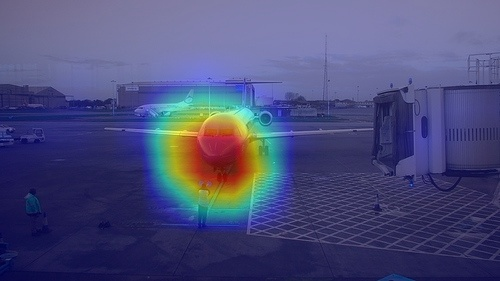Shows `target category` as 417, which corresponds to `ballon` rather than plane.(using the link https://gist.github.com/yrevar/942d3a0ac09ec9e5eb3a

Question:

• Is it something which is generally seen,Since it depends upon what the network is seeing?
• If not, is there a different interpretation of the same result

Regards, Nitin Bansal

opened by nbansal90 10
• #### Bug report!

Greetings, homie!

Like `heatmap = np.float32(heatmap) / 255`, `np.float32(img)` should be devided by 255.0 as well! https://github.com/jacobgil/pytorch-grad-cam/blob/87c1a7c9951a986fbcde89b9a7f946f6e04bf0f8/pytorch_grad_cam/utils/image.py#L45

opened by MarcusNerva 9
• #### Require grad error in Tutorial EigenCAM for YOLO5

Hello, I have tryed your tutorial EigenCAM for yolo5 but I got an error below `RuntimeError: element 0 of tensors does not require grad and does not have a grad_fn` pointing to line `grayscale_cam = cam(tensor)[0, :, :]` and inner line 82 in file base_cam.py: `loss.backward(retain_graph=True)` I'm not sure why this error occured because I just run your code without any modification. It seems that some tensors are not required grad, so it can not go backward. Any help would be greatly appreciated.

opened by zhenyu-brice-zhao 8
• #### HiResCAM : counting 0-value attributions maps in GAP and no-GAP networks

I have trained two variations of an EfficientNet-B2 network on the following dataset.

• Network 1: One structured as “conv layers - GAP layer - raw class scores - softmax” (i.e. as downloaded from PyTorch torchvision plus softmax)
• Network 2: One structured as “conv layers - Flatten - raw class scores - softmax” (i.e. I have removed the GAP layer and have flattened the output of the last conv layer as suggested in the HiResCAM paper)

The networks are comparable in terms of generalization ability yielding similar classification report on the test set (consists of 10% data per class and 2119 images it total). In both networks I applied HiResCAM on the correctly classified test images and my findings are as follows :

• Network 1: 1997/2119 test accuracy and 8/1997 attribution maps have only 0 values
• Network 2: 1971/2119 test accuracy and 537/1971 attribution maps have only 0 values

In other words, it seems that replacing GAP with Flattening has a great influence on the quality of the produced maps, as Network 2 produces almost 500 more 0-valued maps.

Is there an intuition/explanation on this ?

``````test_dataset = ...  #loaded via custom code

gap_instance = HiResCAM(model=effnetb2_gap, target_layers=[effnetb2_gap.features[-1]], use_cuda=False)
flatten_instance = HiResCAM(model=effnetb2_flatten , target_layers=[effnetb2_flatten .features[-1]], use_cuda=False)

a,b = 0,0
c,d = 0,0

for image, label in test_dataset:

if int(torch.argmax(effnetb2_gap(image.unsqueeze(0))))==label:
a+=1
b+=1

if int(torch.argmax(effnetb2_flatten(image.unsqueeze(0))))==label:
c+=1
d+=1

print('GAP - {}/{}'.format(b,a))        # gives  8/1997
print('Flatten - {}/{}'.format(d,c))    # gives 537/1971
``````

Network 1 is as follows :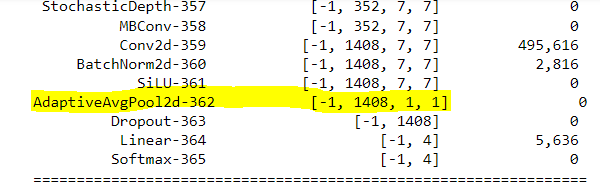Network 2 is as follows :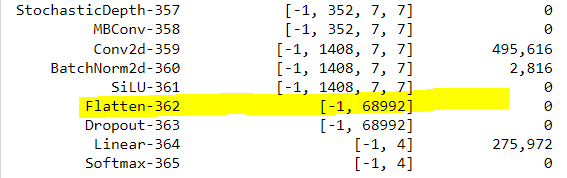opened by vggls 0
• #### AssertionError of FullGrad for the inception_v3 model

For the `inception_v3` model in `torchvision.models`, FullGrad attribution arises the AssertionError about "assert(len(self.bias_data) == len(grads_list))"; I find that the `len(self.bias_data)` is 96 while `len(grads_list)`is just 94 when steps into the functions.

It is just from the normal-usage of the function,

model = torchvision.models.inception_v3(weights=models.Inception_V3_Weights.IMAGENET1K_V1) fg = FullGrad(model, [], use_cuda=True) # FullGrad will ignore the given target_layers, so here it is an empty list attr = fg(input_tensor=x.to(device), targets=[ClassifierOutputTarget(tar_clsidx)])

Does anyone also encounter such a problem? Or any suggestions? @jacobgil

opened by wenchieh 0
• #### References for concept activation maps

I find [Notebook tutorial: Adapting pixel attribution methods for embedding outputs from models] to be useful and very enlightening. However, I would like to go deeper to understand why concept activation maps work. May I ask from what paper it was proposed?

opened by MDK-L 0
• #### Grad-CAM ++ implementation doubts

Hi,

Thanks for all your work for implementing and summarizing the Grad-CAM related methods. It is fun and helpful to understand multiple methods.

I have doubts about the Grad-CAM ++ method, where the equation you are using in your implementation is equation 19 in the paper. However, this equation 19 is only valid for the NN with last activation function as exponential function. It is not the general equation should be used for all NN, e.g. NN with the softmax as last activation function.

I feel the correct implementation should be based on equation 10 of the paper, where the first, second, and third order derivatives are necessary for implementation of general NN. Since equation 19 is only a special case for replacing equation 10 if the last activation is exp.

Thanks.

opened by yangruo1226 0
• #### benchmarking

some scores on different cams (averaged over random 1K images from imagenet val). Insertion (higher is better) and Deletion (lower is better) are metrics that were proposed in the RISE paper. I used ResNet-50 as the model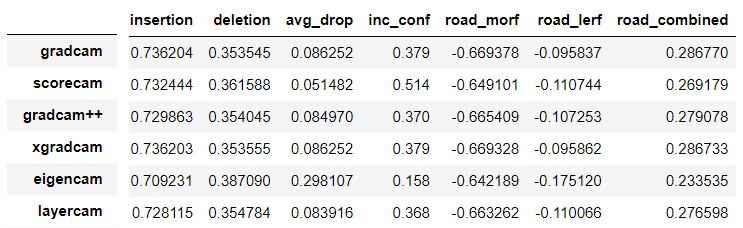opened by fawazsammani 1
###### Jacob Gildenblat
Machine learning / Computer Vision.###### Rational Activation Functions - Replacing Padé Activation Units

Rational Activations - Learnable Rational Activation Functions First introduce as PAU in Padé Activation Units: End-to-end Learning of Activation Func

38 Nov 22, 2022
###### Equivariant CNNs for the sphere and SO(3) implemented in PyTorch

Equivariant CNNs for the sphere and SO(3) implemented in PyTorch

893 Dec 28, 2022
###### Code for paper: Group-CAM: Group Score-Weighted Visual Explanations for Deep Convolutional Networks

Group-CAM By Zhang, Qinglong and Rao, Lu and Yang, Yubin [State Key Laboratory for Novel Software Technology at Nanjing University] This repo is the o

98 Nov 16, 2022
###### Codes for TS-CAM: Token Semantic Coupled Attention Map for Weakly Supervised Object Localization.

TS-CAM: Token Semantic Coupled Attention Map for Weakly SupervisedObject Localization This is the official implementaion of paper TS-CAM: Token Semant

112 Jan 2, 2023
###### Study of human inductive biases in CNNs and Transformers.

Are Convolutional Neural Networks or Transformers more like human vision? This repository contains the code and fine-tuned models of popular Convoluti

39 Dec 8, 2022
###### This repository contains the source code of our work on designing efficient CNNs for computer vision

Efficient networks for Computer Vision This repo contains source code of our work on designing efficient networks for different computer vision tasks:

386 Nov 26, 2022
###### An open source bike computer based on Raspberry Pi Zero (W, WH) with GPS and ANT+. Including offline map and navigation.

Pi Zero Bikecomputer An open-source bike computer based on Raspberry Pi Zero (W, WH) with GPS and ANT+ https://github.com/hishizuka/pizero_bikecompute

264 Jan 2, 2023
###### A PyTorch-based open-source framework that provides methods for improving the weakly annotated data and allows researchers to efficiently develop and compare their own methods.

Knodle (Knowledge-supervised Deep Learning Framework) - a new framework for weak supervision with neural networks. It provides a modularization for se

93 Nov 6, 2022

103 Dec 23, 2022
###### ChatBot-Pytorch - A GPT-2 ChatBot implemented using Pytorch and Huggingface-transformers

ChatBot-Pytorch A GPT-2 ChatBot implemented using Pytorch and Huggingface-transf

42 Dec 9, 2022
###### Contrastive Learning for Many-to-many Multilingual Neural Machine Translation(mCOLT/mRASP2), ACL2021

Contrastive Learning for Many-to-many Multilingual Neural Machine Translation(mCOLT/mRASP2), ACL2021 The code for training mCOLT/mRASP2, a multilingua

104 Jan 1, 2023
###### M2MRF: Many-to-Many Reassembly of Features for Tiny Lesion Segmentation in Fundus Images

M2MRF: Many-to-Many Reassembly of Features for Tiny Lesion Segmentation in Fundus Images This repo is the official implementation of paper "M2MRF: Man

12 Dec 14, 2022
###### An integration of several popular automatic augmentation methods, including OHL (Online Hyper-Parameter Learning for Auto-Augmentation Strategy) and AWS (Improving Auto Augment via Augmentation Wise Weight Sharing) by Sensetime Research.

An integration of several popular automatic augmentation methods, including OHL (Online Hyper-Parameter Learning for Auto-Augmentation Strategy) and AWS (Improving Auto Augment via Augmentation Wise Weight Sharing) by Sensetime Research.

45 Dec 8, 2022
489 Jan 7, 2023
###### aka "Bayesian Methods for Hackers": An introduction to Bayesian methods + probabilistic programming with a computation/understanding-first, mathematics-second point of view. All in pure Python ;)

Bayesian Methods for Hackers Using Python and PyMC The Bayesian method is the natural approach to inference, yet it is hidden from readers behind chap

25.1k Jan 2, 2023
###### Implementation of temporal pooling methods studied in [ICIP'20] A Comparative Evaluation Of Temporal Pooling Methods For Blind Video Quality Assessment

Implementation of temporal pooling methods studied in [ICIP'20] A Comparative Evaluation Of Temporal Pooling Methods For Blind Video Quality Assessment

5 Sep 16, 2022
###### Callable PyTrees and filtered JIT/grad transformations => neural networks in JAX.

Equinox Callable PyTrees and filtered JIT/grad transformations => neural networks in JAX Equinox brings more power to your model building in JAX. Repr

909 Dec 30, 2022
###### PyTorch reimplementation of the Smooth ReLU activation function proposed in the paper "Real World Large Scale Recommendation Systems Reproducibility and Smooth Activations" [arXiv 2022].

Smooth ReLU in PyTorch Unofficial PyTorch reimplementation of the Smooth ReLU (SmeLU) activation function proposed in the paper Real World Large Scale

10 Jan 2, 2023